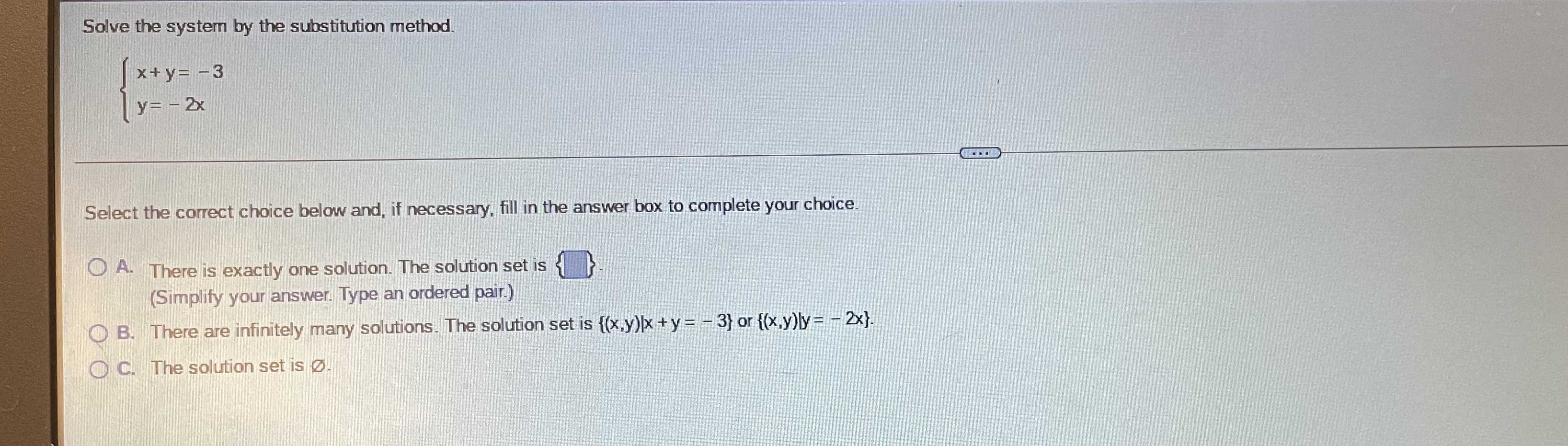### Still have math questions?

Algebra
QuestionSolve the system by the substitution method. $$\left\{ \begin{array} { l } { x + y = - 3 } \\ { y = - 2 x } \end{array} \right.$$ Select the correct choice below and, if necessary, fill in the answer box to complete your choice.

A. There is exactly one solution. The solution set is $$\{ \}$$ . (Simplify your answer. Type an ordered pair.) B. There are infinitely many solutions. The solution set is $$\{ ( x , y ) | x + y = - 3 \}$$ or $$\{ ( x , y ) | y = - 2 x \}$$ .

C. The solution set is $$\varnothing$$ .

$$x= 3,\space y= -6$$